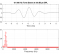## DF2T IIR SOSStarted by 3 years ago2 replieslatest reply 3 years ago205 views

Hi everybody,

i have to write a matlab function to calculate the signal and the snr in output from the Figure 1 circuit (direct form 2 transposed second-order-sections IIR filter).

The function takes in input the filter coefficients (zeros and poles vectors B and A), the signal x[n] and the quantization noise e[n].

The signal x[n] is supposed to be a 44.1kHz vocal audio, while the quantization noise e[n] is a zero mean uniform noise which power is a 1/1000 of x[n]'s power.

B and A are the coefficients of a low pass IIR filter with the following specifications:

- maximum allowed transition band: 200Hz

- cut-off frequency 8kHz

- minimum Astop=70dB

Using filterDesigner toolbox and setting elliptic IIR and minimum order I obtained a 14th-order filter which SOS is a 7x6 matrix

I wrote the circuit function in this way, but i think that i've made some mistakes in its calculation because the signal in output is almost fully covered by noise.

function [y,snr_dB,P_ey_dB] = funzione1(B,A,x,e)

%ritardo massimo

R=length(A);

L=length(x);

v = zeros(L,R);

y = zeros(L,1);

e_y = zeros(L,1);

for k=R+2:length(x)

for m=R-1:-1:1

v(k,m)=B(m+1)*x(k)-A(m+1)*y(k)+v(k-1,m+1);

e_y(k)=e_y(k)-A(m+1)*e_y(k-1);

end

y(k)=B(1)*x(k)+e(k)+v(k-1,1);

e_y(k)=e_y(k)+e(k);

end

P_sig = sum(y.^2)/length(y);

P_noise = sum(e_y.^2)/length(e_y);

P_ey_dB=10*log10(P_noise);

snr=P_sig/P_noise;

snr_dB=10*log10(snr);

end

Figure 2: x[n] spectrogram

Figure 3: y[n] spectrogram

Figure 4: filtered signal using filter function directly non considering quantization noise

The main is:

x=resample(data,441,320);

fs=441*fs/320;

%prendo solamente i primi 3sec della traccia audio

x=x(1:96000);

audiowrite('AudioSample.wav',x/max(abs(x)),44100);

figure('Name','Analisi spettrale del segnale in ingresso');

spectrogram(x,2048,1024,2048,fs);

P_ing=sum(x.^2)/length(x);

P_ing_dB=10*log10(P_ing);

e=0+sqrt(P_ing/1000)*rand(length(x),1);

P_e=sum(e.^2)/length(e);

P_e_dB=10*log10(P_e);

snr_ing_dB=10*log10(P_ing/P_e);

[B,A]=sos2tf(SOSiir,Giir);

[y,snr_out_dB,P_ey_dB]=funzione1(B,A,x,e);

figure('Name','Analisi spettrale del segnale in uscita');

spectrogram(y,2048,1024,2048,fs);

figure;

spectrogram(filter(B,A,x+e),2028,1024,2048,fs);

Can anyone do a quick check, please?

Thanks and sorry for my english. :)

EDIT: 04/04/2020 6:16pm

[ - ]At first glance it appears your "function [y,snr_dB,P_ey_dB] = funzione1(B,A,x,e)" does work properly.  I added a statement at the beginning of this function that zeros the noise vector (i.e. e = zeros(size(e));).  With this change, the spectrums of the filtered signals generated using the FILTER function and your function are similar.

I would step through your function to double-check its implementation.

I hope this helps.

[ - ]Hello Mr. Fragavetti,

here is a MATLAB script that I used to edit an audio file. You need to change the script for your needs.

Always look at the time signals. Instead of the spectrograms, you would have to do a wavelet analysis.

#############################

% Skript audio_1.m

clear;

S = load('handel.mat');     % Data in S.y and sample freq in S.Fs

S

% ------- Write audiosignal in wav File

audiowrite('handel.wav',S.y,S.Fs);     % wav File

player = audioplayer(S.y,S.Fs);

play(player);

pause

figure(1);

plot((0:length(S.y)-1)/S.Fs, S.y);

La = axis;    axis([La(1:2),-1,1]);

title('handel');   xlabel('s');    grid on;

% ------- IIR-Filter

[B,A] = ellip(14,0.1,70,800/(S.Fs/2));   % IIR-Filter

nfft = 512;

HB = fft(B,nfft);

HA = fft(A,nfft);

Hiir = HB./HA;

figure(2);

plot((0:nfft-1)*S.Fs/nfft, 20*log10(abs(Hiir)),'k-','LineWidth',1);

title('Amplitude response of the IIR-Filter in dB')

xlabel('Hz');   grid on;

% -------- Signal plus noise

ny = length(S.y);

%noise = sqrt(std(S.y)^2/1000);       % Variance of noise

noise = sqrt(std(S.y)^2/10);       % Variance of noise

y1 = S.y + noise*randn(ny,1);         % Signal + noise

player = audioplayer(y1,S.Fs);

play(player);

pause;

figure(3);

plot((0:length(y1)-1)/S.Fs, y1,'k-','LineWidth',1);

hold on;

plot((0:length(S.y)-1)/S.Fs, S.y,'r-','LineWidth',1);

La = axis;    axis([La(1:2),-1,1]);

title('Signal and signal with noise');   xlabel('s');    grid on;

% ------ Filtering the Signal

y2 = filter(B,A,y1);

player = audioplayer(y2,S.Fs);

play(player);

pause

figure(4);

subplot(211), plot((0:length(y1)-1)/S.Fs, y1,'r-','LineWidth',1);

title('The signal with noise');

subplot(212), plot((0:length(y2)-1)/S.Fs, y2,'k-','LineWidth',1);

title('The filtered signal');

% ------- Power spectral density

[Py, w] = pwelch(S.y, hamming(512),,,S.Fs,'twosided');

[Py1, w] = pwelch(y1, hamming(512),,,S.Fs,'twosided');

[Py2, w] = pwelch(y2, hamming(512),,,S.Fs,'twosided');

figure(5);

subplot(311), plot(w, 10*log10(Py),'k-','LineWidth',1);

title('Poer spectral density of the original signal');

xlabel('Hz');  axis tight;

subplot(312), plot(w, 10*log10(Py1),'k-','LineWidth',1);

title('Poer spectral density of the signal with noise');

xlabel('Hz');  axis tight;

subplot(313), plot(w, 10*log10(Py2),'k-','LineWidth',1);

title('Poer spectral density of the filtered signal');

xlabel('Hz');  axis tight;

#######################################

audio_1.m